# New to Qlik Sense

Discussion board where members can get started with Qlik Sense.

Announcements
Qlik® Product Spotlight: Discover what’s possible. Get more from our products.
See for yourself. Register today.
Contributor

## cumulative sum in bar chart

Hello everyone,

I am trying to calculate cumulative sum in a bar chart in Qlik Sense which has two dimensions; month and year. My measure is sum of Sales.

What I want is, sales values should be cumulatively summed in bar chart for the selected months.

For example: March 2016  sales values should be sum of Jan 2016 and Feb 2016 on pivot table and rest of them also should be the same.

Right now I have below bar chart, it is only showing the related month year sales data.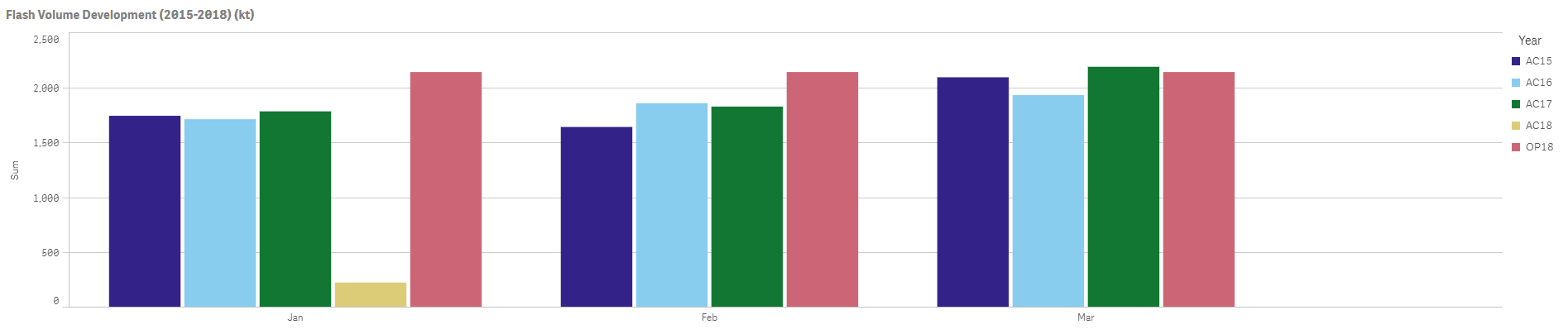I have tried "=RangeSum(Above(Sum(Sales), 0, RowNo(total)))" but it has not worked. Result of the "=RangeSum(Above(Sum(Sales), 0, RowNo(total)))" is below picture.Please suggest me any solution if anyone of you gone through the same problem.

Thanks.

OY

1 Solution

Accepted SolutionsMVP

## Re: cumulative sum in bar chart

Give this a shot

Aggr(RangeSum(Above(Sum(Sales), 0, RowNo())),Year, (Month, (NUMERIC)))

29 Replies
Esteemed Contributor

## Re: cumulative sum in bar chart

try:

aggr(rangesum(above(Sum(Sales),0,RowNo())),SecondDim,FirstDim)

Result (per analogy):

aggr(rangesum(above(Sum(Sales),0,RowNo())),nom,num)Contributor

## Re: cumulative sum in bar chart

Hello Omar,

provided script not working, result of the script is first picure, however month figures should  increase incremant like second picture. related month figures should be cumulatively summed.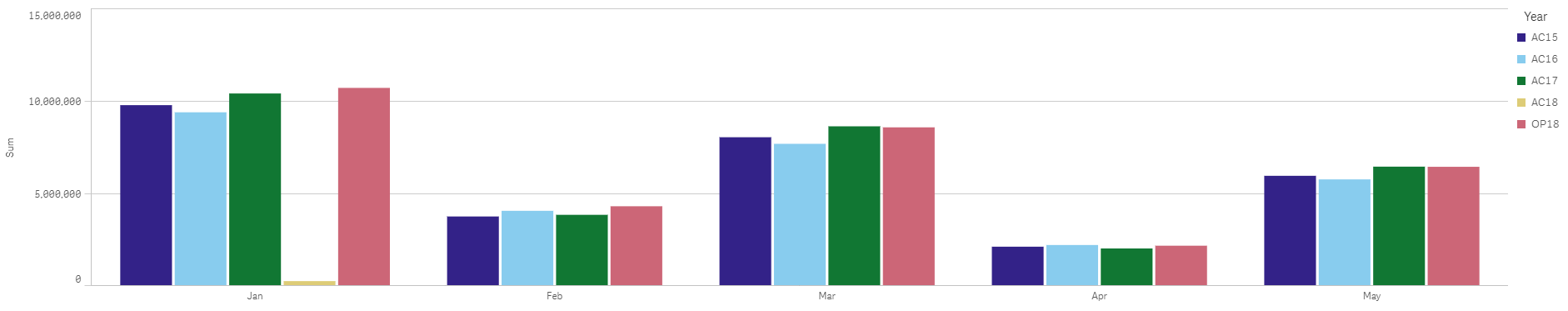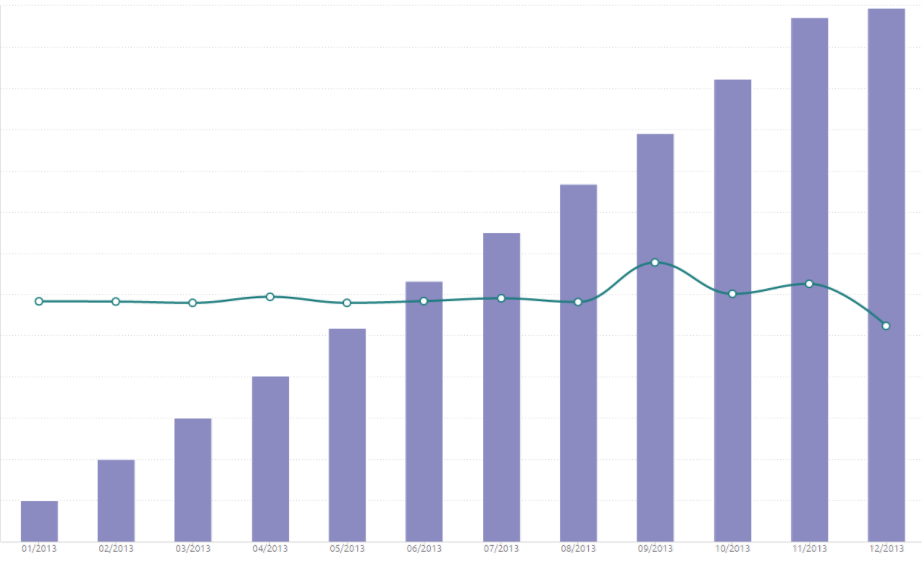Esteemed Contributor

## Re: cumulative sum in bar chart

you want to eliminate the second dimension when u cumulate ur measure?

Because, the way things are with my expressions; each month; the second dimension will be cumulated..

see a and b :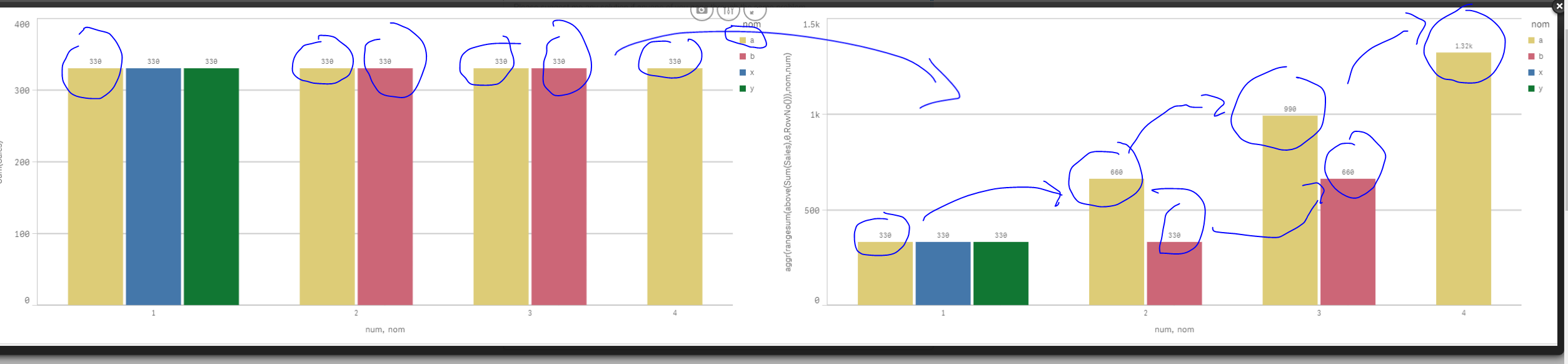if u want to cumulate the whole thing by month and sum up all ur values of ur second dim then do as follow:

aggr(rangesum(above(Sum(Sales),0,RowNo())),Month)

result: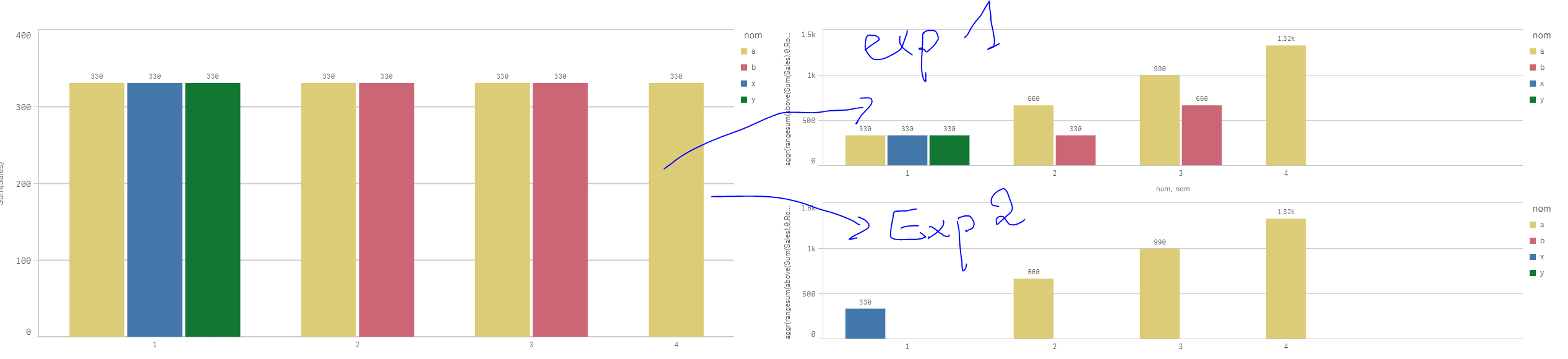Contributor

## Re: cumulative sum in bar chart

I want to cumulate the whole thing, if month Oct is selected, result should be like below picture.

aggr(rangesum(above(Sum(Sales),0,RowNo())),Month) is not working, result is the second pic.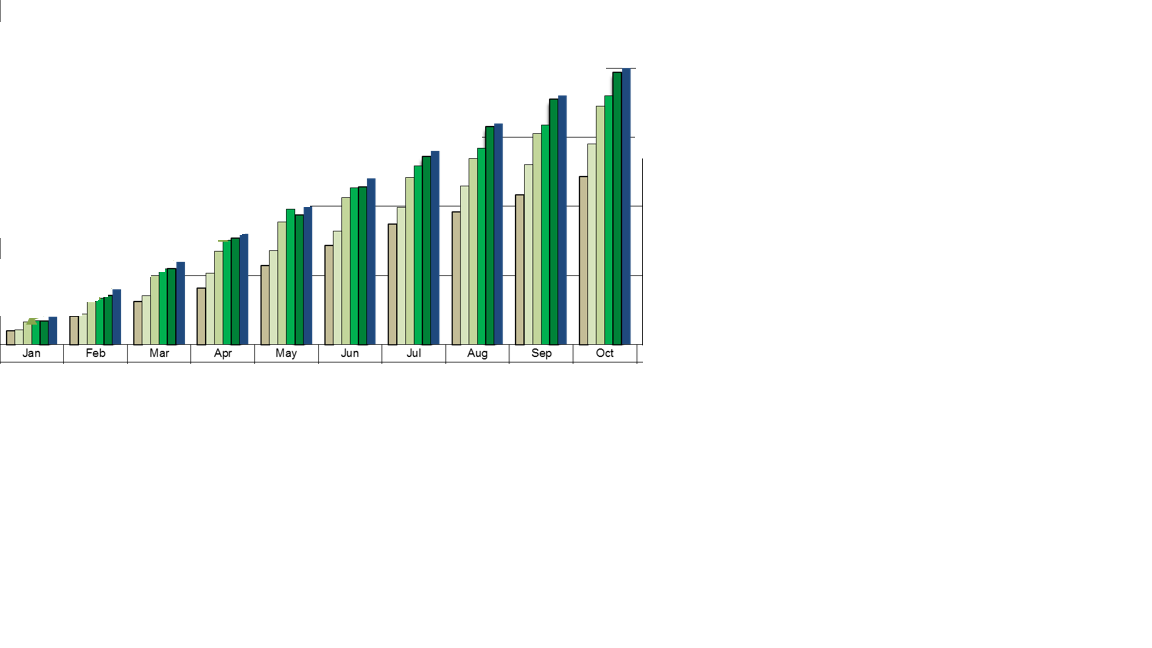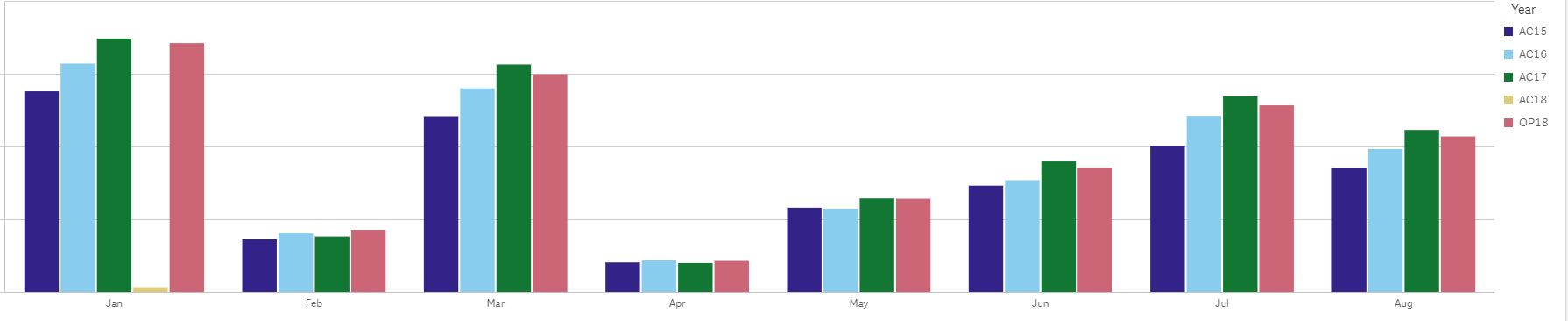Esteemed Contributor

## Re: cumulative sum in bar chart

can u please share a test application so I can take a look at this?

Because it works for me (without selections..)

stalwar1‌, how can this work with selections?

because when I select a month, qlik will this only this month and thus would not be able the above months..

Any idea?Esteemed Contributor

## Re: cumulative sum in bar chart

and please try to also refer to these :

Accumulations

The As-Of TableMVP

## Re: cumulative sum in bar chart

I think Omar is right, have you tried this?

Aggr(RangeSum(Above(Sum(Sales), 0, RowNo())),Year, Month)MVP

## Re: cumulative sum in bar chart

This

Only(<Month, Year>)Aggr(RangeSum(Above(Sum({<Month, Year>}Sales), 0, RowNo())),Year, Month))MVP

## Re: cumulative sum in bar chart

or this

Aggr(RangeSum(Above(Sum({<Month, Year>}Sales), 0, RowNo())),Year, Month)

Community Browser